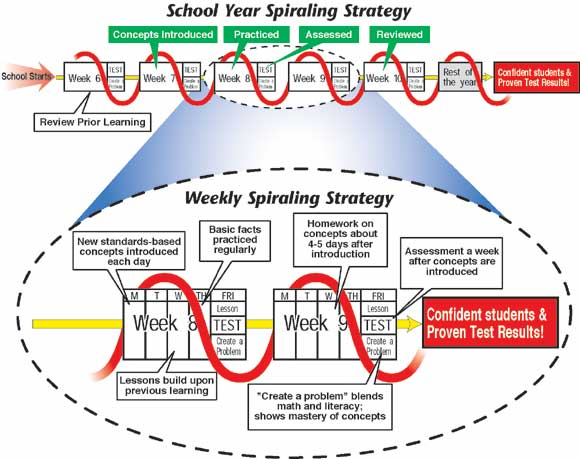## Monday, November 26, 2012

### Fibonacci's Spiral and Excel Math

Today we'll take a look at the Fibonacci spiral and rectangle. Fibonacci, or more correctly Leonardo da Pisa (also called Leonardo Bigollo), was born in Pisa, Italy in 1175. He travelled widely in Barbary (Algeria).

In 1200 he returned to Pisa and used the knowledge he had gained on his travels to write Liber abaci.Fibonacci: 810 Years of Mathematical Magic and
Celebrating Fibonacci Day

The famous Fibonacci sequence is as follows:

1, 1, 2, 3, 5, 8, 13, 21, 34, 55, 89, 144, 233, 377, 610, 987, ...

where the first two numbers of the series are 1 and 1 and each number afterward is defined as the sum of the two previous terms, Fn = Fn - 2 + Fn - 1. (Though in Fibonacci's sequence the first number was 1 and the second number was two, the first one was assumed.)
We can make a picture showing the Fibonacci numbers 1, 1, 2, 3, 5, 8, 13, 21... if we start with two small squares of size 1 next to each other. On top of both of these draw a square of size 2 (= 1 + 1).

We can now draw a new square—touching both a unit square and the latest square of side 2—so having sides 3 units long; and then another touching both the 2-square and the 3-square (which has sides of 5 units). We can continue adding squares around the picture, each new square having a side which is as long as the sum of the latest two square's sides. This set of rectangles whose sides are two successive Fibonacci numbers in length and which are made up of squares with sides which are Fibonacci numbers, we will call the Fibonacci Rectangles.Here is a spiral drawn in the squares, a quarter of a circle in each square. The spiral is is made up of fragments which are parts of circles and does not go on getting smaller and smaller) but it is a good approximation to a kind of spiral that does appear often in nature. Such spirals are seen in the shape of shells of snails and sea shells and in the arrangement of seeds on flowering plants. The spiral-in-the-squares makes a line from the centre of the spiral increase by a factor of the golden number in each square. So points on the spiral are 1.618 times as far from the centre after a quarter-turn. In a whole turn the points on a radius out from the centre are 1.6184 = 6.854 times further out than when the curve last crossed the same radial line.

This golden ratio 1·618034 is also called the golden section or the golden mean or just the golden number. It is often represented by a Greek letter Phi. The closely related value which we write as phi with a small "p" is just the decimal part of Phi, namely 0·618034.This is an image of a Nautilus sea shell. You can see the spiral curve of the shell. The internal chambers that the animal using it adds on as it grows. The chambers provide buoyancy in the water. Now draw an imaginary line from the center of the shell out in any direction and find two places where the shell crosses it so that the shell spiral has gone round just once between them. The outer crossing point will be about 1.6 times as far from the centre as the next inner point on the line where the shell crosses it. This shows that the shell has grown by a factor of the golden ratio in one turn.This mathematical spiral can also be seen in music. According to a recent article in the Wall Street Journal, Debussy worked in spirals. "Musicologists have detected evidence of spiral-like mathematical structures in this music, related to the Fibonacci sequence and the Golden Mean."

Read more at Ron Knott's web site: Fibonacci Numbers and the Golden Section.

Excel Math uses a spiraling strategy similar to that found in the nautilus shell. This unique spiraling strategy introduces new math concepts to students while reviewing previously-taught concepts. It gives students the opportunity to master the old through spaced repetition, while being challenged with the new. Once a concept is introduced, it literally stays in front of the students for the rest of the school year. The spiraling strategy of repeating concepts at regular intervals throughout the curriculum is an integral part of Excel Math.

This spiraling strategy is a sophisticated process of introducing new concepts, reinforcing the concepts regularly, and then assessing the concepts. It leads to mastery and long-term competency for each student. In other words, the spiraling strategy helps move new concepts into the child's long-term memory and keep them there. Read more on our previous blog post: Spiraling Into Control.

Excel Math curriculum continually brings in new topics while refreshing math concepts the students have learned before. Students aren't tested on a subject until they've had multiple chances to succeed in Guided Practice and Homework. Here's a visual road map explaining this spiraling strategy:Constant review and spaced repetition of the math concepts ensure students remember those concepts long after they are first introduced. Lessons build upon previous learning and often blend math and literacy, producing well-rounded and confident students. To learn more, visit the Excel Math website.